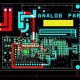# What is Flip flop & Its Types?

In this tutorial, we are going to learn about Flip – Flop & its types.

Flip – Flop:

It is a basic digital memory circuit or one bit memory cell which can store one bit of information & It has  two outputs that’s  are complement to each other and  it acts as a bistable multivibrator which  has two stable stage 0 and 1. When the circuit is in one state then continues to remain in that state it is called as memory. While this information is locked or latched, therefore flip flop is also called as latch.

Types of Flip – Flop:

1. SR Latch:  It is also called Set Reset Latch which affects the outputs as long as the enable, E is maintained at ‘1’. Below is the circuit diagram of SR Latch.

As per above circuit has two inputs which are S and R and also has two outputs Q (t) & Q (t). The upper NOR gate has two inputs R & complement of present state, Qtt’ and produces next state, Qt+1t+1 then enable, E is ‘1’.

Same like, the lower NOR gate has two inputs S & present state, Qtt and produces complement of next state, Qt+1t+1’ when enable, E is ‘1’.& a 2-input NOR gate produces an output that is the complement of another input when one of the input is ‘0’. Same as, it is produce ‘0’ output, that time one of the input is ‘1’.

• When S = 1, then next state Qt+1t+1 will be equal to ‘1’ irrespective of present state, Qtt values.
• When R = 1, then next state Qt+1t+1 will be equal to ‘0’ irrespective of present state, Qtt values.

Generally, only of those two inputs should be ‘1’. If both inputs are ‘1’, then the next state Qt+1t+1 value is undefined.

The following table shows the state table of SR latch.

Therefore, SR Latch performs three types of functions such as Hold, Set & Reset based on the input conditions.

• D Latch:  This is also called as Data Latch & below is the circuit diagram of D Latch.

As per above circuit has single input D and two outputs Qtt and Qtt’ and D Latch is coming from SR Latch by placing an inverter between S and R inputs and connect D input to S. It is the combinations of S & R are of same value.

• If D = 0 → S = 0 & R = 1, then next state Qt+1t+1 will be equal to ‘0’ irrespective of present state, Qtt values. It is corresponding to the second row of SR Latch state table.
• If D = 1 → S = 1 & R = 0, then next state Qt+1t+1 will be equal to ‘1’ irrespective of present state, Qtt values. It is corresponding to the third row of SR Latch state table.

Below is table shows the state table of D latch.

While, D Latch Hold the information which is available on data input, “D” which means the output of D Latch is sensitive to the changes in the input, “D” as long as the enable is high.

3. JK Flip-Flop: This is the modified version of SR flip-flop which is operates with only positive clock transitions or negative clock transitions. Below is the circuit  of JK flip-flop.

As per above circuit has two inputs which is J & K and two outputs which is Qtt & Qtt’. The working of JK flip-flop is same as SR flip-flop. In this circuit, considered the inputs of SR flip-flop as S = J Qtt and R = KQtt in order to utilize the modified SR flip-flop for 4 combinations of inputs.

The below is table which show the state table of JK flip-flop.

As per this Qtt & Qt+1t+1 are present state and next state accordingly. So that, JK flip-flop can be used for one of these four functions which are Hold, Reset, Set and  Complement of present state based on the input conditions, If the positive transition of clock signal is applied. The below is table which shows the characteristic table of JK flip-flop.

By using three variable K-Map, we can get the simplified expression for next state, Qt+1t+1. Three variable K-Map for next state, Qt+1t+1 is showing in below diagram.

The maximum possible groupings of adjacent ones are already shown in the circuit. While, the simplified expression for next state Qt+1t+1 is

Q(t+1)=JQ(t)′+K′Q(t)Q(t+1)=JQ(t)′+K′Q(t)

4.  T Flip-Flop: It is the simplified version of JK flip-flop which is obtained by connecting the same input ‘T’ to both inputs of JK flip-flop. T Flip – Flop  operates with only positive clock transitions or negative clock transitions. Below is the  circuit diagram of T flip-flop.

As per above circuit has single input T and two outputs Qtt & Qtt’. The working of T flip-flop is same as that of JK flip-flop. Now we considered the inputs of JK flip-flop as J = T and K = T in order to utilize the modified JK flip-flop for 2 combinations of inputs. So that, it is eliminated the other two combinations of J & K, for which those two values are complement to each other in T flip-flop.

The below is table which is show the state table of T flip-flop.

And here, Qtt & Qt+1t+1 are present state & next state respectively. So that, T flip-flop can be used for one of these two functions such as Hold and Complement of present state based on the input conditions, If positive transition of clock signal is applied. The Below table which shows the characteristic table of T flip-flop.

As per the above characteristic table, we can directly write the next state equation as below:

Q(t+1)=T′Q(t)+TQ(t)′Q(t+1)=T′Q(t)+TQ(t)′

⇒Q (t+1)=T⊕Q (t)⇒Q(t+1)=T⊕Q(t)

The output of this flip – flop always toggles for every positive transition of the clock signal, when input T remains at logic High 11. While, T flip-flop can be used in counters.